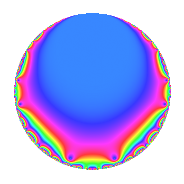# Properties

 Label 600.1.n.aLevel 600 Weight 1 Character orbit 600.n Self dual yes Analytic conductor 0.299 Analytic rank 0 Dimension 1 Projective image $$D_{2}$$ CM/RM discs -15, -24, 40 Inner twists 4

# Related objects

## Newspace parameters

 Level: $$N$$ = $$600 = 2^{3} \cdot 3 \cdot 5^{2}$$ Weight: $$k$$ = $$1$$ Character orbit: $$[\chi]$$ = 600.n (of order $$2$$, degree $$1$$, minimal)

## Newform invariants

 Self dual: yes Analytic conductor: $$0.299439007580$$ Analytic rank: $$0$$ Dimension: $$1$$ Coefficient field: $$\mathbb{Q}$$ Coefficient ring: $$\mathbb{Z}$$ Coefficient ring index: $$1$$ Twist minimal: no (minimal twist has level 120) Projective image $$D_{2}$$ Projective field Galois closure of $$\Q(\sqrt{-6}, \sqrt{10})$$ Artin image $D_4$ Artin field Galois closure of 4.0.9000.1

## $q$-expansion

 $$f(q)$$ $$=$$ $$q - q^{2} + q^{3} + q^{4} - q^{6} - q^{8} + q^{9} + O(q^{10})$$ $$q - q^{2} + q^{3} + q^{4} - q^{6} - q^{8} + q^{9} + q^{12} + q^{16} - q^{18} - q^{24} + q^{27} - 2q^{31} - q^{32} + q^{36} + q^{48} - q^{49} + 2q^{53} - q^{54} + 2q^{62} + q^{64} - q^{72} - 2q^{79} + q^{81} - 2q^{83} - 2q^{93} - q^{96} + q^{98} + O(q^{100})$$

## Character values

We give the values of $$\chi$$ on generators for $$\left(\mathbb{Z}/600\mathbb{Z}\right)^\times$$.

 $$n$$ $$151$$ $$301$$ $$401$$ $$577$$ $$\chi(n)$$ $$1$$ $$-1$$ $$-1$$ $$1$$

## Embeddings

For each embedding $$\iota_m$$ of the coefficient field, the values $$\iota_m(a_n)$$ are shown below.

For more information on an embedded modular form you can click on its label.

Label $$\iota_m(\nu)$$ $$a_{2}$$ $$a_{3}$$ $$a_{4}$$ $$a_{5}$$ $$a_{6}$$ $$a_{7}$$ $$a_{8}$$ $$a_{9}$$ $$a_{10}$$
101.1
 0
−1.00000 1.00000 1.00000 0 −1.00000 0 −1.00000 1.00000 0
 $$n$$: e.g. 2-40 or 990-1000 Significant digits: Format: Complex embeddings Normalized embeddings Satake parameters Satake angles

## Inner twists

Char Parity Ord Mult Type
1.a even 1 1 trivial
15.d odd 2 1 CM by $$\Q(\sqrt{-15})$$
24.h odd 2 1 CM by $$\Q(\sqrt{-6})$$
40.f even 2 1 RM by $$\Q(\sqrt{10})$$

## Twists

By twisting character orbit
Char Parity Ord Mult Type Twist Min Dim
1.a even 1 1 trivial 600.1.n.a 1
3.b odd 2 1 600.1.n.b 1
4.b odd 2 1 2400.1.n.a 1
5.b even 2 1 600.1.n.b 1
5.c odd 4 2 120.1.i.a 2
8.b even 2 1 600.1.n.b 1
8.d odd 2 1 2400.1.n.b 1
12.b even 2 1 2400.1.n.b 1
15.d odd 2 1 CM 600.1.n.a 1
15.e even 4 2 120.1.i.a 2
20.d odd 2 1 2400.1.n.b 1
20.e even 4 2 480.1.i.a 2
24.f even 2 1 2400.1.n.a 1
24.h odd 2 1 CM 600.1.n.a 1
40.e odd 2 1 2400.1.n.a 1
40.f even 2 1 RM 600.1.n.a 1
40.i odd 4 2 120.1.i.a 2
40.k even 4 2 480.1.i.a 2
45.k odd 12 4 3240.1.bh.h 4
45.l even 12 4 3240.1.bh.h 4
60.h even 2 1 2400.1.n.a 1
60.l odd 4 2 480.1.i.a 2
80.i odd 4 2 3840.1.c.a 1
80.j even 4 2 3840.1.c.b 1
80.s even 4 2 3840.1.c.c 1
80.t odd 4 2 3840.1.c.d 1
120.i odd 2 1 600.1.n.b 1
120.m even 2 1 2400.1.n.b 1
120.q odd 4 2 480.1.i.a 2
120.w even 4 2 120.1.i.a 2
240.z odd 4 2 3840.1.c.b 1
240.bb even 4 2 3840.1.c.d 1
240.bd odd 4 2 3840.1.c.c 1
240.bf even 4 2 3840.1.c.a 1
360.br even 12 4 3240.1.bh.h 4
360.bu odd 12 4 3240.1.bh.h 4

By twisted newform orbit
Twist Min Dim Char Parity Ord Mult Type
120.1.i.a 2 5.c odd 4 2
120.1.i.a 2 15.e even 4 2
120.1.i.a 2 40.i odd 4 2
120.1.i.a 2 120.w even 4 2
480.1.i.a 2 20.e even 4 2
480.1.i.a 2 40.k even 4 2
480.1.i.a 2 60.l odd 4 2
480.1.i.a 2 120.q odd 4 2
600.1.n.a 1 1.a even 1 1 trivial
600.1.n.a 1 15.d odd 2 1 CM
600.1.n.a 1 24.h odd 2 1 CM
600.1.n.a 1 40.f even 2 1 RM
600.1.n.b 1 3.b odd 2 1
600.1.n.b 1 5.b even 2 1
600.1.n.b 1 8.b even 2 1
600.1.n.b 1 120.i odd 2 1
2400.1.n.a 1 4.b odd 2 1
2400.1.n.a 1 24.f even 2 1
2400.1.n.a 1 40.e odd 2 1
2400.1.n.a 1 60.h even 2 1
2400.1.n.b 1 8.d odd 2 1
2400.1.n.b 1 12.b even 2 1
2400.1.n.b 1 20.d odd 2 1
2400.1.n.b 1 120.m even 2 1
3240.1.bh.h 4 45.k odd 12 4
3240.1.bh.h 4 45.l even 12 4
3240.1.bh.h 4 360.br even 12 4
3240.1.bh.h 4 360.bu odd 12 4
3840.1.c.a 1 80.i odd 4 2
3840.1.c.a 1 240.bf even 4 2
3840.1.c.b 1 80.j even 4 2
3840.1.c.b 1 240.z odd 4 2
3840.1.c.c 1 80.s even 4 2
3840.1.c.c 1 240.bd odd 4 2
3840.1.c.d 1 80.t odd 4 2
3840.1.c.d 1 240.bb even 4 2

## Hecke kernels

This newform subspace can be constructed as the kernel of the linear operator $$T_{53} - 2$$ acting on $$S_{1}^{\mathrm{new}}(600, [\chi])$$.

## Hecke Characteristic Polynomials

$p$ $F_p(T)$
$2$ $$1 + T$$
$3$ $$1 - T$$
$5$ 1
$7$ $$1 + T^{2}$$
$11$ $$1 + T^{2}$$
$13$ $$( 1 - T )( 1 + T )$$
$17$ $$( 1 - T )( 1 + T )$$
$19$ $$( 1 - T )( 1 + T )$$
$23$ $$( 1 - T )( 1 + T )$$
$29$ $$1 + T^{2}$$
$31$ $$( 1 + T )^{2}$$
$37$ $$( 1 - T )( 1 + T )$$
$41$ $$( 1 - T )( 1 + T )$$
$43$ $$( 1 - T )( 1 + T )$$
$47$ $$( 1 - T )( 1 + T )$$
$53$ $$( 1 - T )^{2}$$
$59$ $$1 + T^{2}$$
$61$ $$( 1 - T )( 1 + T )$$
$67$ $$( 1 - T )( 1 + T )$$
$71$ $$( 1 - T )( 1 + T )$$
$73$ $$1 + T^{2}$$
$79$ $$( 1 + T )^{2}$$
$83$ $$( 1 + T )^{2}$$
$89$ $$( 1 - T )( 1 + T )$$
$97$ $$1 + T^{2}$$